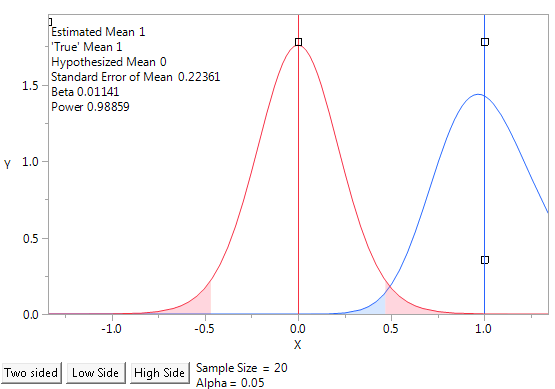Design of Experiments Guide > Prospective Sample Size and Power
Publication date: 08/13/2020

# Prospective Sample Size and Power

Use the Sample Size and Power platform to investigate the impact of sample size on your ability to answer specific questions when planning experiments or studies.

How many units should I test?

Will I be able to detect a difference in my treatment means?

How many units must I test to estimate failure time?

The Sample Size and Power platform is a collection of calculators. The calculators have fields for data entry and calculated values. You can select values to specify and those to calculate. For example, you might specify power and the effect size that you want to detect and then calculate the necessary sample size. Alternatively, you might specify a sample size and power to calculate the effect size that you can detect.

Sample size calculations for hypothesis tests are based on the trade off between Type I and Type II errors. The Type I error, or α, is the probability of rejecting the null hypothesis when it is true. The Type II error, or β, is the probability of not rejecting the null hypothesis when it is false. Generally you would like α and β to be small. Power, or 1 - β, is the probability of rejecting the null hypothesis when it is false.

Figure 17.1 Power Animation for One Sample Mean